Browse by Topic
Related Topics

# Falling Faster than the Speed of Sound

Earlier this month, on a nice day, Felix Baumgartner jumped from 39,045 meters, or 24.26 miles, above the Earth from a capsule lifted by a 334-foot-tall helium filled balloon (twice the height of Nelson’s column and 2.5 times the diameter of the Hindenberg). Wolfram|Alpha tells us the jump was equivalent to a fall from 4.4 Mount Everests stacked on top of each other, or falling 93% of the length of a marathon.

At 24.26 miles above the Earth, the atmosphere is very thin and cold, only about -14 degrees Fahrenheit on average. The temperature, unlike air pressure, does not change linearly with altitude at such heights. As Wolfram|Alpha shows us, it rises and falls depending on factors such as the decreased density of air with rising altitude, but also the absorption of UV light by the ozone layer.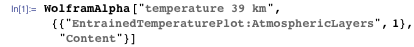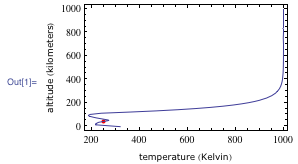At 39 kilometers, the horizon is roughly 439 miles away. At this layer of the atmosphere, called the stratosphere, the air pressure is only 3.3 millibars, equivalent to 0.33% of the air pressure at sea level. To put it another way, the mass of the air above 39 kilometers is only 0.32851% of the total air mass. Given this knowledge, we know that 99.67% of the world’s atmosphere lay beneath him. This information was important to Felix’s goal to break the sound barrier in free fall because the rate of drag is directly related to air pressure. With less air around him, there would be less drag, and thus he could reach a higher maximum speed. Of course, this would require him to wear an oxygenated suit to allow him to breathe, in addition to keeping him warm.

The fact that the speed of sound varies with altitude also helped. At high altitudes, because of the cold and the low density of the atmosphere, it takes longer for sound to travel. This is not a linear relationship, as the temperature and chemical makeup of the atmosphere varies significantly with altitude, and also affects the speed of sound: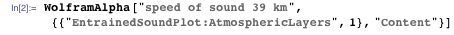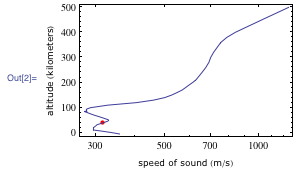We can zoom in on the area of interest with some short Mathematica code. Here we just extract the plot points, adjusting for the logarithm taken for our x coordinates and flipping the x and y axes.Taking the interpolation, we can get a closer look at the relevant portions of Felix’s jump.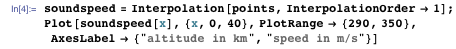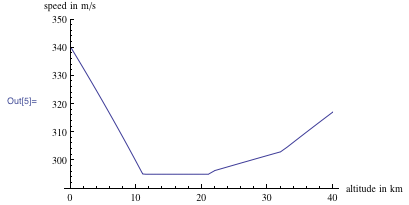We can see that there is a nice minimum for the speed of sound in the middle of Felix’s fall, ideal if one wants to break the sound barrier.

So how easy is it to break the sound barrier by falling? Wolfram|Alpha can’t yet easily tell us, but with a bit of help from Mathematica, we can find out.

First we need to examine the equations of motion. Air resistance is dominated by the quadratic term for high velocities and takes the form: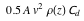where v is velocity, Cd is the drag coefficient, A is maximal cross-sectional area, and ρ(z) is air density at altitude z. The other major force working on our jumper is gravity g. Now the acceleration due to gravity doesn’t vary very much over the range the jump: 9.69 meters per second when he jumps to 9.831 meters per second when he lands. This isn’t surprising, since relative to the radius of the Earth (3,956.6 miles), 24.26 miles is a short distance. Air density varies a great deal more: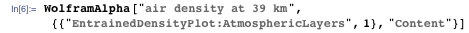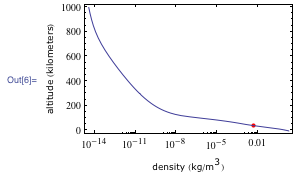Our full equation of motion is: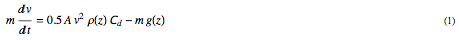where m is the mass of Felix and his gear. We will ignore the added mass effect in our example. The added mass effect accounts for the additional force required to move the surrounding fluid, in this case air, around a moving object. It typically adds to the effective mass of an object in the equation. This mass is based on the volume of the falling object, which given Felix’s relative size and the extremely low density of the atmosphere, is likely to be small in any case.

Let’s rearrange this equation to look at the velocity as a function of altitude: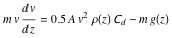With the data we have, we can’t solve this symbolically, but we can derive a numerical solution. First we define ρ(z) and g(z). As before, we can extract the air density data from Wolfram|Alpha: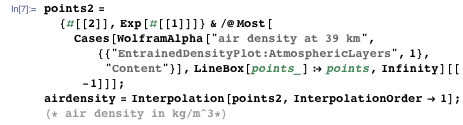Whereas gravity we can determine from the standard gravitational formula: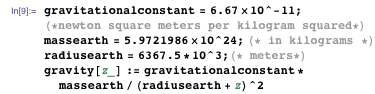Next we make some assumptions about the other physical parameters. Felix’s mass is unknown, especially with all of his gear needed to survive at those altitudes, but a normal human mass is around 60 kg. Assuming a large amount of gear, we might guess a mass of 90 kg or about 200 lbs.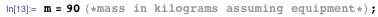Next we need a drag coefficient. A calculation of supersonic fluid dynamics is outside the scope of this blog post, but some elementary research shows that subsonic drag coefficients are considered to be independent of velocity. An upright human (which is not necessarily the case for Felix, who was tumbling for much of his fall) has a drag coefficient between 1 and 1.3. Research with dropped bombs at supersonic speeds suggests that the increased drag of the shock wave can more than double drag. In the end it is hard to gauge his effective drag coefficient under these circumstances. Let’s assume he has a drag toward the upper end of the normal (subsonic) range, and hits supersonic speeds for only a short period.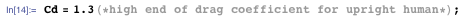Cross-sectional area is another complicated affair. If Felix was rigidly upright, this might be as low as a square foot or 0.1 square meters (assuming average shoulder width and body depth). But according to reports, he did go into a spin that might have increased his cross section to be lengthwise, and thus upwards of 7 square feet or 0.7 square meters. We’ll split the difference.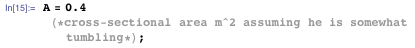Before we look at how his speed changed through his descent, we might also look at what the maximum free fall velocity is. In that case, equation (1) would look like: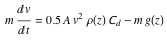since velocity is unchanging. We can rearrange the terms to solve for v:Let’s put this all together and see how his fall progressed.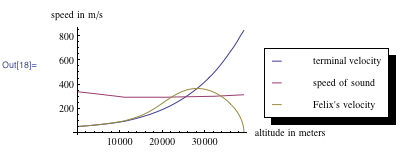What we see is that Felix’s speed (in yellow) rapidly increases until it meets or exceeds the speed of sound (in purple) around 30,000 meters. It also briefly exceeds terminal velocity (in blue), increasing his instability as the atmosphere decelerates him.

We can divide his velocity by the speed of sound at that altitude to find his local Mach number: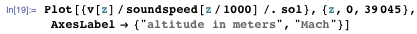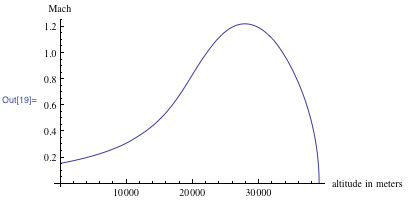We can also use Mathematica to find where the maximum occurs.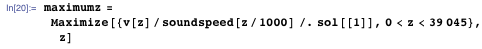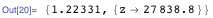Compared to official reports of about 30,000 meters, we are off by a couple kilometers, likely due to an inaccurate accounting for the drag coefficient at supersonic speeds. Our maximum value, however, is more encouraging and close to current official results of Mach 1.24.

Running this code several more times for different heights, we can see that Felix needed to jump from higher than 33,000 meters in order to achieve supersonic speeds.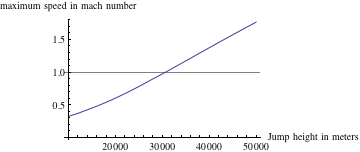Or seen another way, how his speed would have changed depending on his initial height: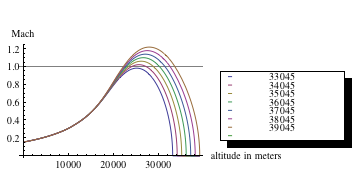Meanwhile, the total time of his fall can be found from the velocity function. Since v = dz/dt, we can rearrange the equation as dz/v = dt: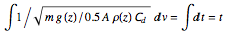We integrate from his jump height to the time of his parachute deployment at around 2,500 meters.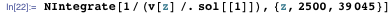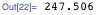This yields a time of 4 minutes 8 seconds, which is close to the actual time of 4 minutes 22 seconds. This loss might be due to our assumptions for the drag coefficient. With a little adjustment, we might try to crudely account for the change in the drag coefficient, Cd, based on relative velocity: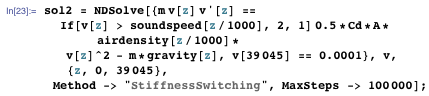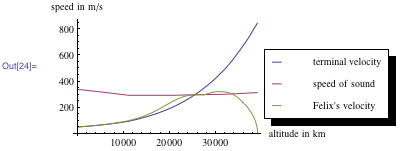This sharply localizes his supersonic travel as the increased drag quickly slows him down. This adds a few seconds to his flight time: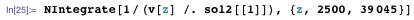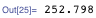Which brings the published values of Felix’s jump and the result of our model in closer agreement.

Returning to our original solution, what if he had failed to open his parachute?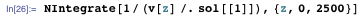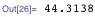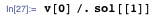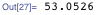He would have fallen for roughly another 44 seconds, possibly breaking the record for the longest time for free fall. Of course he would have also slammed into the Earth at 53 meters per second, or over 118 miles per hour. Probably not worth it.

The tumble that happened to Felix could have easily caused that tragic event to happen, though. But how can that sort of instability develop? For this we might look at Euler’s equations. If we treat Felix like a rigid box or cuboid, we find that the moment of inertia tensor for a cuboid is: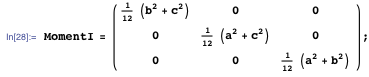Making some simple assumptions about our jumper’s dimensions, we set (in meters):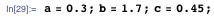If we imagine that in the course of his fall, Felix is given a small perturbation so that his angular velocity is now:

ω = Ω1 x + Ω2 y + nz

where x, y, and z are unit vectors, then our initial conditions are: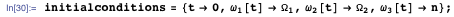Euler’s equations are (the ωi are the body-fixed angular velocities):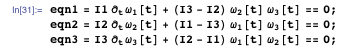where: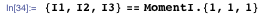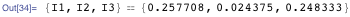If ω1 and ω2 are very small relative to the partial derivative in time t of ω3, then ω3 will be approximately constant. From this we can see that if we eliminate ω2 from the first two equations, we get: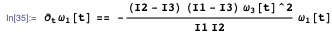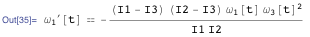Here we can see that if I3 is either the largest or smallest moment of inertia, then the coefficient for ω1 is negative, and this is the equation for a simple harmonic oscillator. Thus the motion is stable. If however I3 has the middle value, then this leads to unstable motion.

How unstable? To get a quantitative feel, the following interactive demonstration shows clearly that an initial main rotation about the second axis quickly causes much more substantial rotations around the first axis than vice versa.As can be seen, the motion is erratic, rotating significantly along every axis, and is a very dangerous position to be in. Fortunately Felix was able to arrest the spin and get himself under control so that he could deploy his parachute properly. It was an exciting spectacle, and one for the history books.